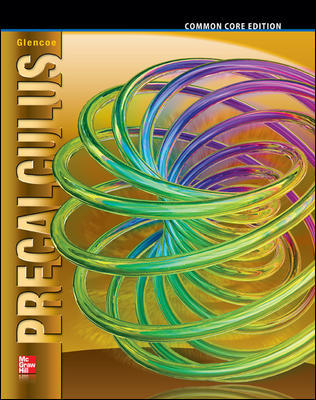### PrecalculusIn Self-paced Precalculus, students learn the fundamental precalculus topics needed to succeed in Calculus. This course systematically covers all the typical material from a high school precalculus course, featuring:

• Dedicated Lecture Videos: 30+ hours of dedicated videos covering key concepts and example problems for each section.
• Homework Assignments:  Practice problems to build and strengthen knowledge for each section.
• Chapter Exams: Test student understanding with multiple choice (timed) exams.

Tuition for the course is \$980 and includes access to all the recorded session and homework. The required textbook below is not included. The course covers chapters 1 through 10. •Required Textbook: Glencoe Precalculus. The textbook is required for assigned readings and practice for the homework. The book is available for rent or purchase through McGraw Hill. ### Graded Self-paced Precalculus (Transcript Available)In Self-paced Precalculus, students learn the fundamental precalculus topics needed to succeed in Calculus. This course systematically covers all the typical material from a high school precalculus course, featuring: • Dedicated Lecture Videos: 30+ hours of dedicated videos covering key concepts and example problems for each section. • Homework Assignments: Practice problems to build and strengthen knowledge for each section. • Chapter Exams: Test student understanding with multiple choice (timed) exams. In the graded version of the course, students submit homework assignments and receive feedback from instructors. A final grade and course transcript are provided upon successful completion of the course. The final grade is based on student performance on homework, chapter tests, and a final exam. Tuition for the course is$1650. The required textbook below is not included. The course covers chapters 1 through 10.

•Required Textbook: Glencoe Precalculus. The textbook is required for assigned readings and practice for the homework. The book is available for rent or purchase through McGraw Hill.